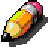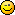You are not logged in.

• Index
•  » General Help
•  » GRRLIB_NPlot :: How to use it an how to dinamically set the Vector?

## #1 2009-06-26 22:45:15

Member

### GRRLIB_NPlot :: How to use it an how to dinamically set the Vector?

Hi, I need to draw a graph showing the x position of the wiimote in function of the time.

The (simpified) code should be something like this:

#### Code:

```33        if ( held & WPAD_BUTTON_A ){
34            graph[grr]={grr, ir1.sx, 0};
35            grc++;
36            GRRLIB_NPlot(graph, 0xFFFFFFFF, 640);
37            if(grc==640)grc=0;
38        }```

(Of course each variable and the vector are properly defined in main() ).

But it doesn't work, I get this error when compiling:

#### Code:

`main.c:34: error: expected expression before '{' token`

What am I doing wrong? I couldn't find any example using it, so I have no reference, and the only example using vectors (Day 9 tutorial) defines the vector at main(), and I need to define it in while(1).

Thanks previously.

Offline

## #2 2009-06-26 23:11:40

Crayon### Re: GRRLIB_NPlot :: How to use it an how to dinamically set the Vector?

Hi, graph is declared as what? Could paste the code?

Offline

## #3 2009-06-26 23:14:19

Member

### Re: GRRLIB_NPlot :: How to use it an how to dinamically set the Vector?

What I really need is to show a graph of the distance from the screen center to the wiimote cursor.

#### Code:

```#include <stdio.h>
#include <stdlib.h>
#include <gccore.h>
#include <math.h>
#include "GRRLIB/GRRLIB/GRRLIB.h"
#include "gfx/console.h"

Mtx GXmodelView2D;

int main() {

ir_t ir1;

// Initialise the video system
GRRLIB_Init();

// This function initialises the attached controllers

GRRLIB_texImg tex_font = GRRLIB_LoadTexture(console);
GRRLIB_InitTileSet(&tex_font, 8, 8, 0);

int grc = 0;
Vector grafica;

while(1) {

u32 pressed = WPAD_ButtonsDown(0);
u32 held = WPAD_ButtonsHeld(0);

int xpos = ir1.sx-250;
int ypos = ir1.sy-250;

// Si pulsamos A calculamos la distancia del cursor al centro de la pantalla y trazamos la línea que une a este con el cursor
if ( held & WPAD_BUTTON_A ){
int vx = abs(xpos-320)^2;
int vy = abs(ypos-240)^2;
int distance = (vx+vy);
GRRLIB_Printf(10, 10, tex_font, 0xFFFFFFFF,1, "\n%i", distance);
GRRLIB_Printf(10, 20, tex_font, 0xFFFFFFFF,1, "\n%i", grc);
GRRLIB_Line(320, 240, xpos, ypos, 0xFFFFFFFF);    //vector hacia el lápiz
grc++;
grafica[grc]={grc, distance, 0.0f}
GRRLIB_NPlot(grafica, 0xFFFFFFFF, 640);
if(grc==640)grc=0;
}

// We return to the launcher application via exit
if ( pressed & WPAD_BUTTON_HOME ) break;

GRRLIB_Render();
}
GRRLIB_Exit();
return 0;
}```

Last edited by Kadede (2009-06-26 23:14:57)

Offline

## #4 2009-06-26 23:25:37

Crayon### Re: GRRLIB_NPlot :: How to use it an how to dinamically set the Vector?

Ok I don't know the type Vector? And what is the Day 9 tutorial?

Offline

## #5 2009-06-26 23:51:28

Member

### Re: GRRLIB_NPlot :: How to use it an how to dinamically set the Vector?

This is the day 9 tutorial:
http://grrlib.santo.fr/wiki/wikka.php?wakka=Day9

Of course, it works if I give the vector values in main(), but this is not what I want. In adittion, I need 640 different vectors - too much to be defined.Last edited by Kadede (2009-06-26 23:59:59)

Offline

## #6 2009-06-27 00:12:47

Crayon### Re: GRRLIB_NPlot :: How to use it an how to dinamically set the Vector?

This is the day 9 tutorial:
http://grrlib.santo.fr/wiki/wikka.php?wakka=Day9

This an old GRRLIB 3.0.1 alpha tutorial.

Would it be easier to create an array of struct?

#### Code:

```typedef struct
{
int Count;
int Position;
int Something;
} MyArray;

MyArray grafica;```

I not sure yet I understand what you want to do!

Offline

## #7 2009-06-27 00:19:29

NoNameNo

### Re: GRRLIB_NPlot :: How to use it an how to dinamically set the Vector?

hi Crayon,

From what i know, Vector is something like this :

typedef struct
{
int x;
int y;
int z;
} Vector;

so...

Offline

## #8 2009-06-27 00:23:03

Crayon### Re: GRRLIB_NPlot :: How to use it an how to dinamically set the Vector?

#### NoNameNo wrote:

hi Crayon,

From what i know, Vector is something like this :

typedef struct
{
int x;
int y;
int z;
} Vector;

so...

It's almost thatThat's what I just found in gu.h

#### Code:

```typedef struct _vecf {
f32 x,y,z;
} Vector;```

Offline

## #9 2009-06-27 00:36:18

Member

### Re: GRRLIB_NPlot :: How to use it an how to dinamically set the Vector?

Crayon and Nonameno, really thanks for the help. Finally I solved it by this way:

#### Code:

```            grc++;
grafica[grc]=distance;
for(i=0; i<640; i++){
GRRLIB_Plot(i, grafica[i], 0xFFFFFFFF);
}
if(grc==640)grc=0;```

It is simplier and easier. Thanks again.

EDIT:

This way better:

#### Code:

```
grc++;
grafica[grc]=480-distance;
for(i=0; i<640; i++){
if(grafica[i]!=0)GRRLIB_Line(i, grafica[i], i-1, grafica[i+-1], 0x00FF00FF);
}
if(grc==640)grc=0;```

Last edited by Kadede (2009-06-27 00:49:58)

Offline

## #10 2009-06-27 00:52:17

Crayon### Re: GRRLIB_NPlot :: How to use it an how to dinamically set the Vector?

The other way around would have been:

#### Code:

```            Vector vTemp;
vTemp.x = grc;
vTemp.y = distance;
vTemp.z = 0.0f;
grafica[grc] = vTemp;
GRRLIB_NPlot(grafica, 0xFFFFFFFF, 640);```

Offline

## #11 2009-07-07 22:12:20

Crayon### Re: GRRLIB_NPlot :: How to use it an how to dinamically set the Vector?

#### Crayon wrote:

That's what I just found in gu.h

#### Code:

```typedef struct _vecf {
f32 x,y,z;
} Vector;```

19 hours ago the Vector structure was change to guVector to avoid collisions.
Changes could be seen on this page: http://devkitpro.svn.sourceforge.net/vi … mp;r2=3650

Offline

## #12 2009-07-08 09:32:06

NoNameNo

### Re: GRRLIB_NPlot :: How to use it an how to dinamically set the Vector?

according to this i think grrlib need a little update...

Offline

• Index
•  » General Help
•  » GRRLIB_NPlot :: How to use it an how to dinamically set the Vector?